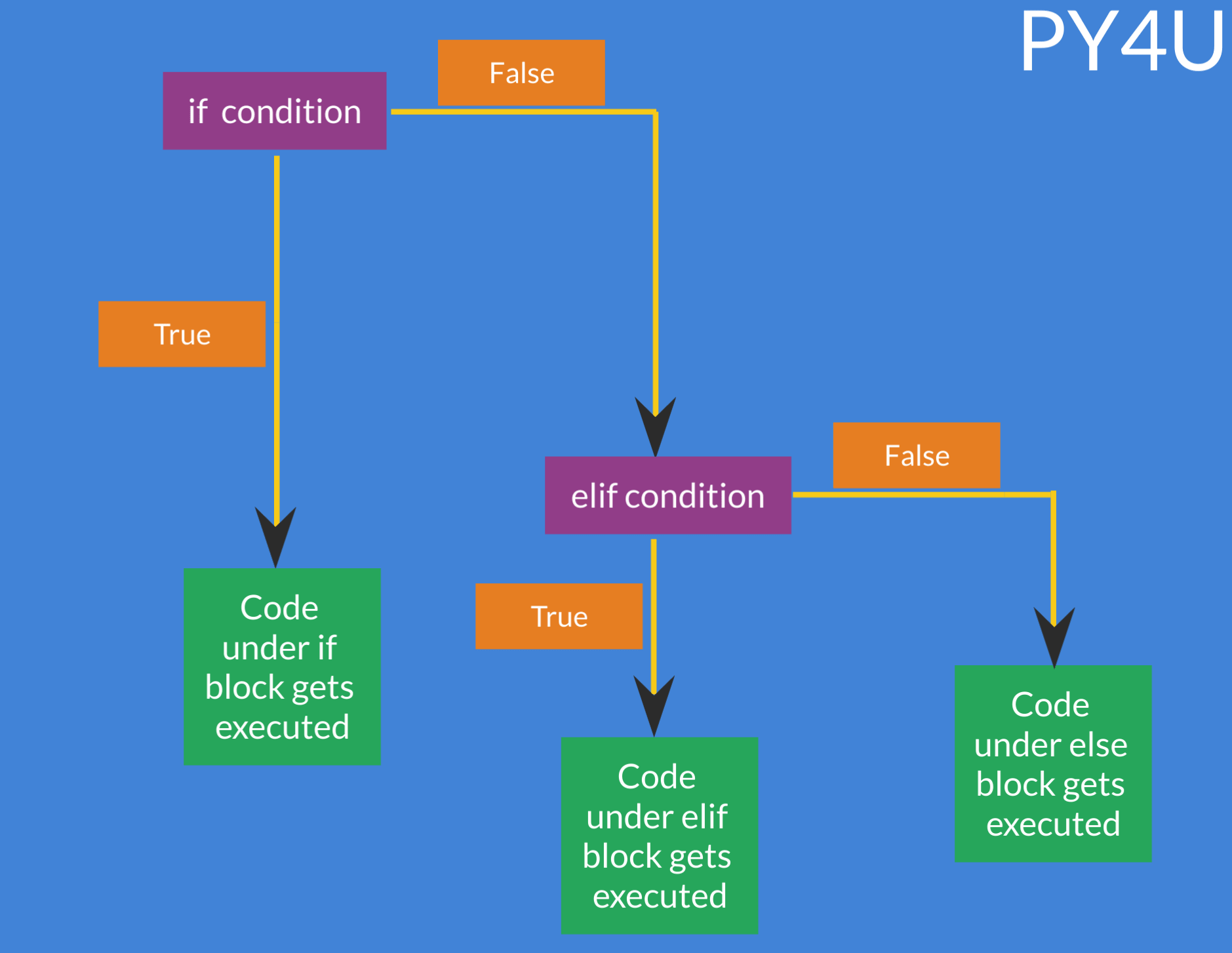Conditional Statements In Python

Conditional statements decides if a section of the programme will be executed or not based upon the fulfilment of certain condition(s). This is called Control flow . There are three types of conditional statements :

• if
• elif
• else

Conditional statements in Python make use of 'colons' and indentation ( whitespaces ) . This indentation system is very crucial to Python and is what sets it apart from other languages.The flowchart above explains how the three conditional statements work together to manage the flow of logic .If the condition in the if statement turns out to be true , then the code inside that particular if statement will get executed and elif , else statements will not be executed. If the condition in if statement turns out to be false , then elif statement , if true , will be executed . if both of them ( if and elif) are false , then and only then the else statement will be executed.

Let us quickly go through the syntax of each conditional statement.

• if Some_condition :
# some action
• elif Some_other_condition :
# some action
• else :
# some actionYou need not worry about the indentation in Python , After writing colon , once you press enter the cursor will automatically align itself. Most ide's ( integrated development environment) and text editors ( that supports .py scripts) will support this feature.

Let us go ahead and take a few examples .

if 5>1 :
print("PY4U is an amazing site")
elif 5<1 :
print("this will not run since if is true")
else :
print("this will not run")
output :
PY4U is an amazing site
if 5>1 :
print("this will run")
if 4>2 :
print("this will also run")
else :
print("this will not run")
output :
this will run
this will also run

Note that at times you'll need a condition that is always true , so for that you can simply use the True boolean value in the following way :

• if True :
# the code within this block will always get executed

To make sure that a condition is always false such that block of code within that conditional statement is never executed , use the False boolean value :

• if False :
# the code within this block will never be executed

Let us see this with help of an example.

if True :
print("this will always be executed")
if False :
print("this is never be executed")
a = "hello"
if "hello" == a:
print("== is an equals to sign")
output :
this will always be executed
== is an equals to sign
Nested conditional statements

Nested conditional statements means one or more than one conditional statements within a parent conditional statement . Nested conditional statements are mostly used to break a big and complex programme into multiple parts/sections in a structured way. Let us take an example to understand this.

Consider a problem where we take an input from the user and if that number is even and more than 5 , then print 'yes' and if that number is odd and less then 5 then print 'no'. However if none of the above condition is satisfied then print 'none'.

my_num = int(input('Please enter the number : '))

# block for even numbers
if my_num % 2 ==0:
if my_num > 5 :
print("yes")
else :
print("none")

# block for odd
if my_num % 2 !=0:
if my_num < 5 :
print("no")
else :
print("none")
input :
7
output :
none

Note that for the above example , using nested conditional statement is not an optimized solution , instead 3 separate conditional statements would have been enough . Let us see the above example without nested conditional statements.

my_num = int(input())
if my_num % 2 == 0 and my_num > 5 :
print("yes")
elif my_num % 2 !=0  and my_num < 5 :
print("no")
else :
print("none")

Popular Tutorials